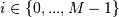numpy.linalg.eig¶

numpy.linalg.eig(a)[source]

Compute the eigenvalues and right eigenvectors of a square array.

Parameters: a : (..., M, M) array Matrices for which the eigenvalues and right eigenvectors will be computed w : (..., M) array The eigenvalues, each repeated according to its multiplicity. The eigenvalues are not necessarily ordered. The resulting array will be of complex type, unless the imaginary part is zero in which case it will be cast to a real type. When a is real the resulting eigenvalues will be real (0 imaginary part) or occur in conjugate pairs v : (..., M, M) array The normalized (unit “length”) eigenvectors, such that the column v[:,i] is the eigenvector corresponding to the eigenvalue w[i]. LinAlgError If the eigenvalue computation does not converge.

eigvals
eigenvalues of a non-symmetric array.
eigh
eigenvalues and eigenvectors of a symmetric or Hermitian (conjugate symmetric) array.
eigvalsh
eigenvalues of a symmetric or Hermitian (conjugate symmetric) array.

Notes

New in version 1.8.0.

Broadcasting rules apply, see the numpy.linalg documentation for details.

This is implemented using the _geev LAPACK routines which compute the eigenvalues and eigenvectors of general square arrays.

The number w is an eigenvalue of a if there exists a vector v such that dot(a,v) = w * v. Thus, the arrays a, w, and v satisfy the equations dot(a[:,:], v[:,i]) = w[i] * v[:,i] for.

The array v of eigenvectors may not be of maximum rank, that is, some of the columns may be linearly dependent, although round-off error may obscure that fact. If the eigenvalues are all different, then theoretically the eigenvectors are linearly independent. Likewise, the (complex-valued) matrix of eigenvectors v is unitary if the matrix a is normal, i.e., if dot(a, a.H) = dot(a.H, a), where a.H denotes the conjugate transpose of a.

Finally, it is emphasized that v consists of the right (as in right-hand side) eigenvectors of a. A vector y satisfying dot(y.T, a) = z * y.T for some number z is called a left eigenvector of a, and, in general, the left and right eigenvectors of a matrix are not necessarily the (perhaps conjugate) transposes of each other.

References

G. Strang, Linear Algebra and Its Applications, 2nd Ed., Orlando, FL, Academic Press, Inc., 1980, Various pp.

Examples

>>> from numpy import linalg as LA

(Almost) trivial example with real e-values and e-vectors.

>>> w, v = LA.eig(np.diag((1, 2, 3)))
>>> w; v
array([ 1.,  2.,  3.])
array([[ 1.,  0.,  0.],
[ 0.,  1.,  0.],
[ 0.,  0.,  1.]])

Real matrix possessing complex e-values and e-vectors; note that the e-values are complex conjugates of each other.

>>> w, v = LA.eig(np.array([[1, -1], [1, 1]]))
>>> w; v
array([ 1. + 1.j,  1. - 1.j])
array([[ 0.70710678+0.j        ,  0.70710678+0.j        ],
[ 0.00000000-0.70710678j,  0.00000000+0.70710678j]])

Complex-valued matrix with real e-values (but complex-valued e-vectors); note that a.conj().T = a, i.e., a is Hermitian.

>>> a = np.array([[1, 1j], [-1j, 1]])
>>> w, v = LA.eig(a)
>>> w; v
array([  2.00000000e+00+0.j,   5.98651912e-36+0.j]) # i.e., {2, 0}
array([[ 0.00000000+0.70710678j,  0.70710678+0.j        ],
[ 0.70710678+0.j        ,  0.00000000+0.70710678j]])

>>> a = np.array([[1 + 1e-9, 0], [0, 1 - 1e-9]])
>>> # Theor. e-values are 1 +/- 1e-9
>>> w, v = LA.eig(a)
>>> w; v
array([ 1.,  1.])
array([[ 1.,  0.],
[ 0.,  1.]])

numpy.linalg.svd

Next topic

numpy.linalg.eigh